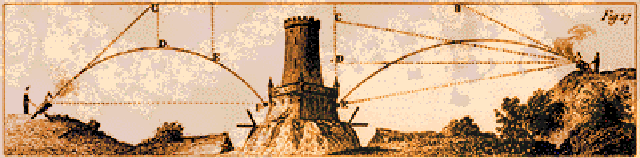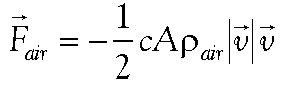PHYS 551 TERM PROJECT

Due: 12/15/2000 without fail (or with, if it is late!)You are a mediæval gunner, whose cannon fires a solid lead ball with the parameters given in the Table below. You are in Italy, at 43° N. latitude. Compute the angle of inclination from the horizontal, q, and the direction of aim, f (measured from true North), to hit a target located a distance R=2 km due East of, and 100 m higher than the cannon, with a precision of ±0.5 m.

Take into account the Coriolis effect of the Earth's rotation, as well as air resistance. Include in the latter the effect of the variation of air density with height, and assume the cannon is at sea level.

The formula for (non-viscous) air resistance iswhere A is the projectile's cross-sectional area, c is a shape parameter—about 0.3 for spheres—and rair is the density of air (which may vary—for example, with altitude). The factor ½ is conventional. Quantities with an arrow are 3-vectors and the notation |vector| stands for the length of the corresponding vector quantity. The – sign indicates that the drag force opposes the motion, i.e. it points exactly in the opposite direction to the instantaneous velocity.

The Coriolis force can be found in any decent book on theoretical mechanics, for example Goldstein or Landau & Lifschitz. It is also given in the printed class notes.

Use either an optimizer or a root-finder to determine the optimum aim points. (Note: since the impact point is a function of two variables, a root finder will have to be more complex than those discussed in class. See, e.g., Acton's book.)

With what precision must the aiming angles and the muzzle velocity be known, in order to assure the projectile will pass within 0.5 m of the target? (Note: bear in mind this is a 3-dimensional problem.)

Parameters
v0 = 180 m/s m = 20 kg c = 0.3 g = 9.80665 m/s2